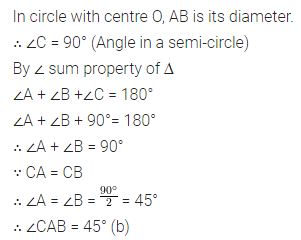## ML Aggarwal Class 8 Solutions for ICSE Maths Chapter 15 Circle Objective Type Questions

Mental Maths
Question 1.
Fill in the blanks:
(i) A chord of a circle is a line segment with its endpoints ……………
(ii) A diameter of a circle is a chord that …………… the centre of the circle.
(iii) A line meets a circle almost in …………… points.
(iv) One-half of the whole arc of a circle is called a …………… of the circle.
(v) The angle subtended by an arc of a circle at the centre of the circle is called the …………… by the arc.
(vi) A line which meets a circle in one and only one point is called a …………… to the circle.
(vii) The tangent at any point of a circle and the radius through that point are …………… to each other.
(viii) From a point outside the circle, …………… tangents can be drawn to the circle.
(ix) The measure of an angle in a semicircle is ……………
Solution: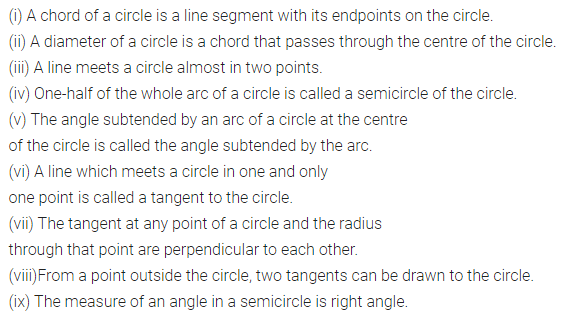Question 2.
State whether the following statements are true (T) or false (F):
(i) A line segment with its end-points lying on a circle is called a radius of the circle.
(ii) Diameter is the longest chord of the circle.
(iii) The end-points of a diameter of a circle divide the circle into two parts; each part is called a semicircle.
(iv) A diameter of a circle divides the circular region into two parts; each part is called a semicircular region.
(v) The diameters of a circle are concurrent. The centre of the circle is the point common to all diameters.
(vi) Every circle has unique centre and it lies inside the circle.
(vii) Every circle has unique diameter.
(viii) From a given point in the exterior of a circle, two tangents can be drawn to it and these two tangents are equal in length.
Solution:Multiple Choice Questions
Choose the correct answer from the given four options (3 to 6):
Question 3.
If P and Q are any two points on a circle, then the line segment PQ is called a
(b) diameter of the circle
(c) chord of the circle
(d) secant of the circle
Solution: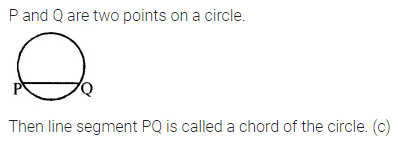Question 4.
If P is a point in the interior of a circle with centre O and radius r, then
(a) OP = r
(b) OP > r
(c) OP > r
(d) OP < r
Solution:Question 5.
If AB = 12 cm, BC = 16 cm and AB is perpendicular to BC, then the radius of the circle passing through the points A, B and C is
(a) 6 cm
(b) 8 cm
(c) 10 cm
(d) 20 cm
Solution: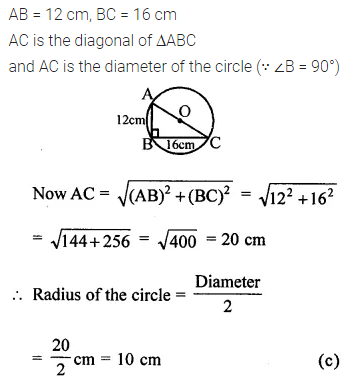Question 6.
In the given figure, AB is a diameter of the circle. If AC = BC, then ∠CAB is equal to
(a) 30°
(b) 45°
(c) 60°
(d) 90°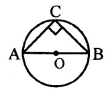Solution: